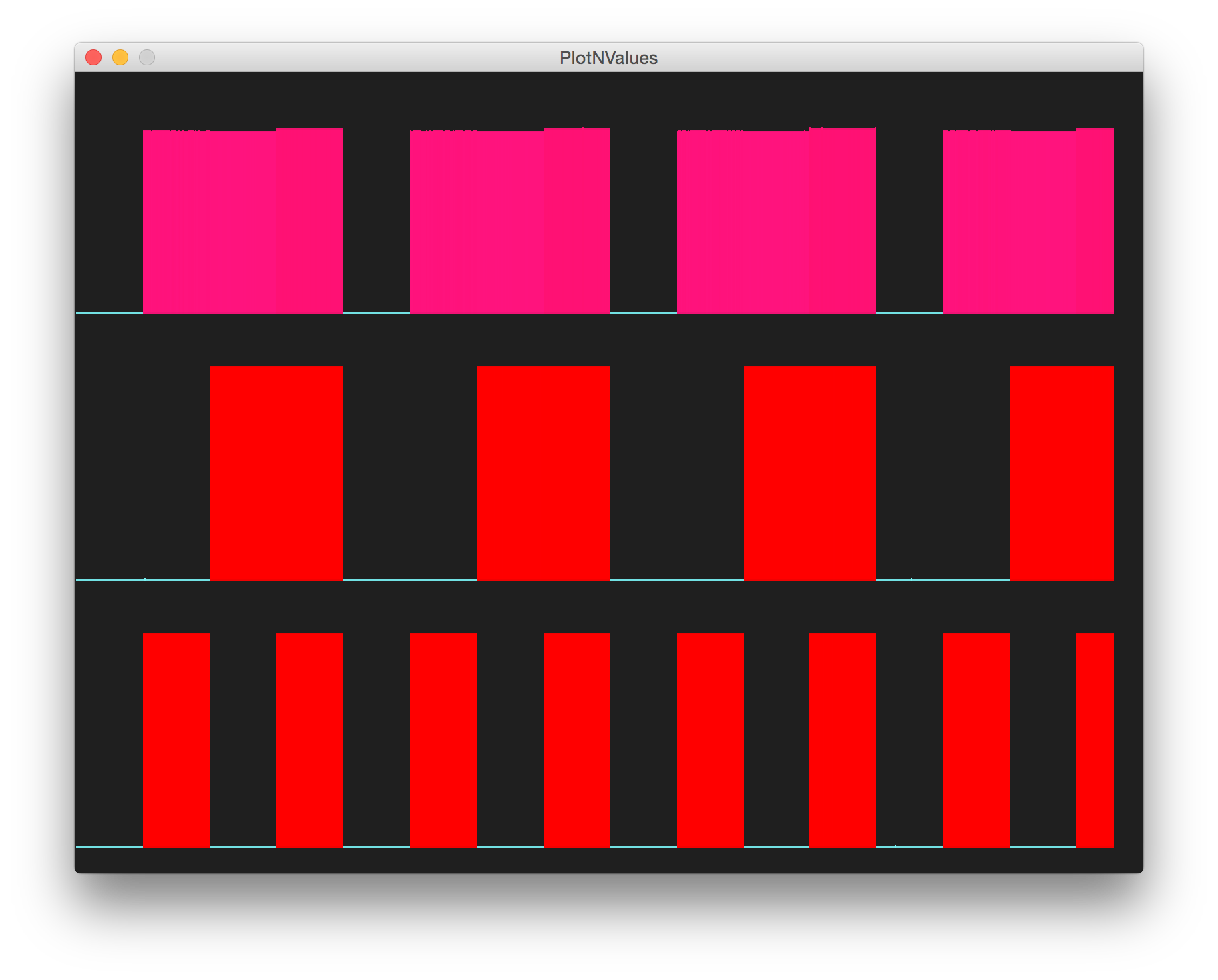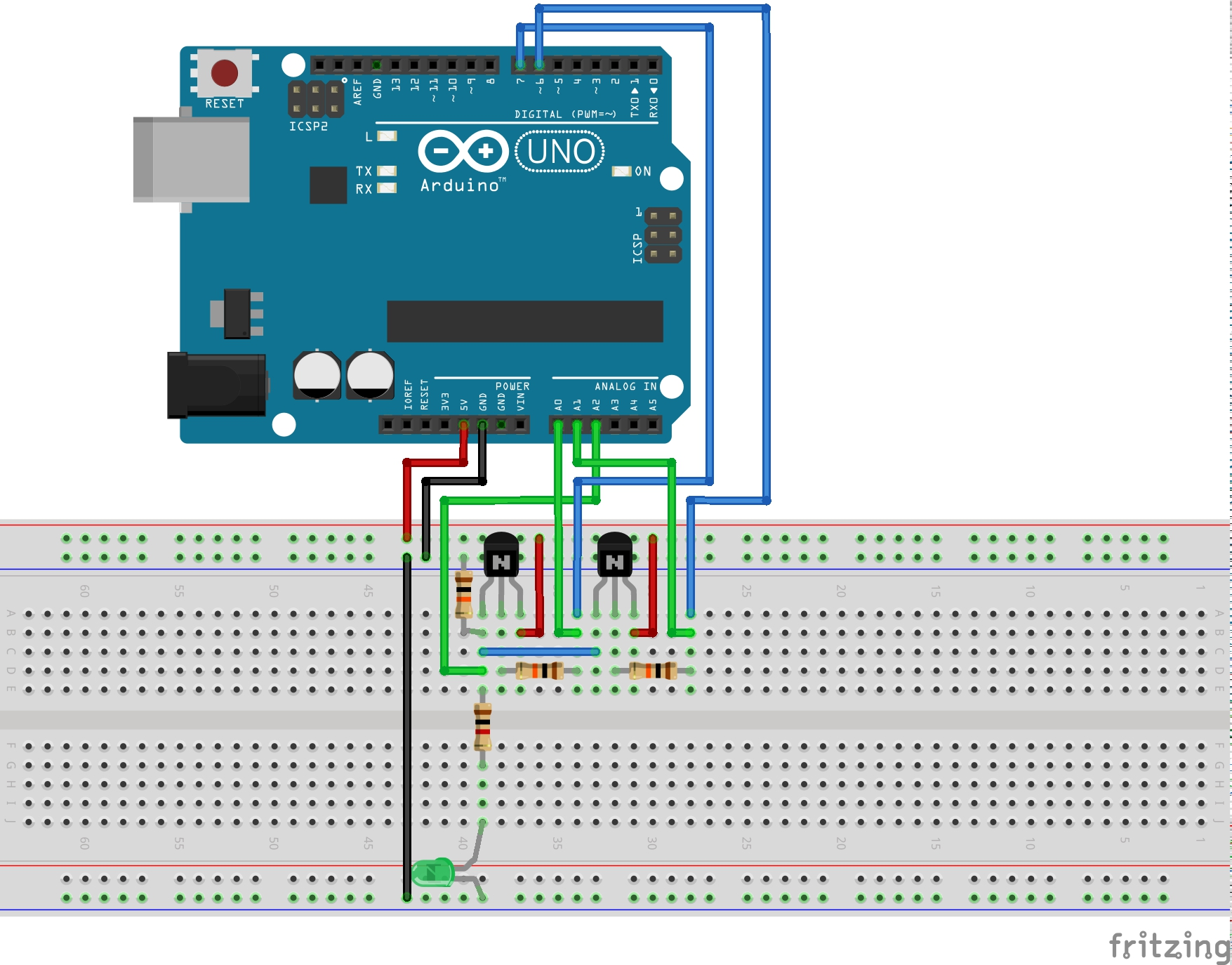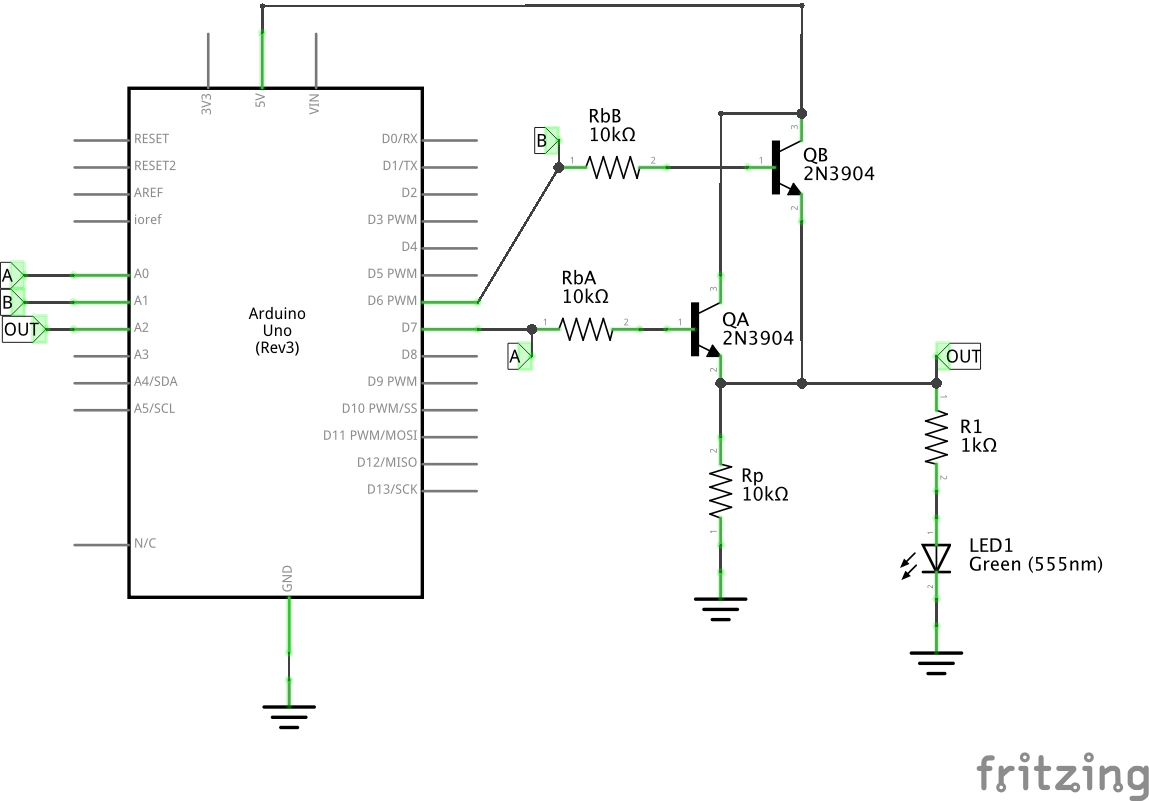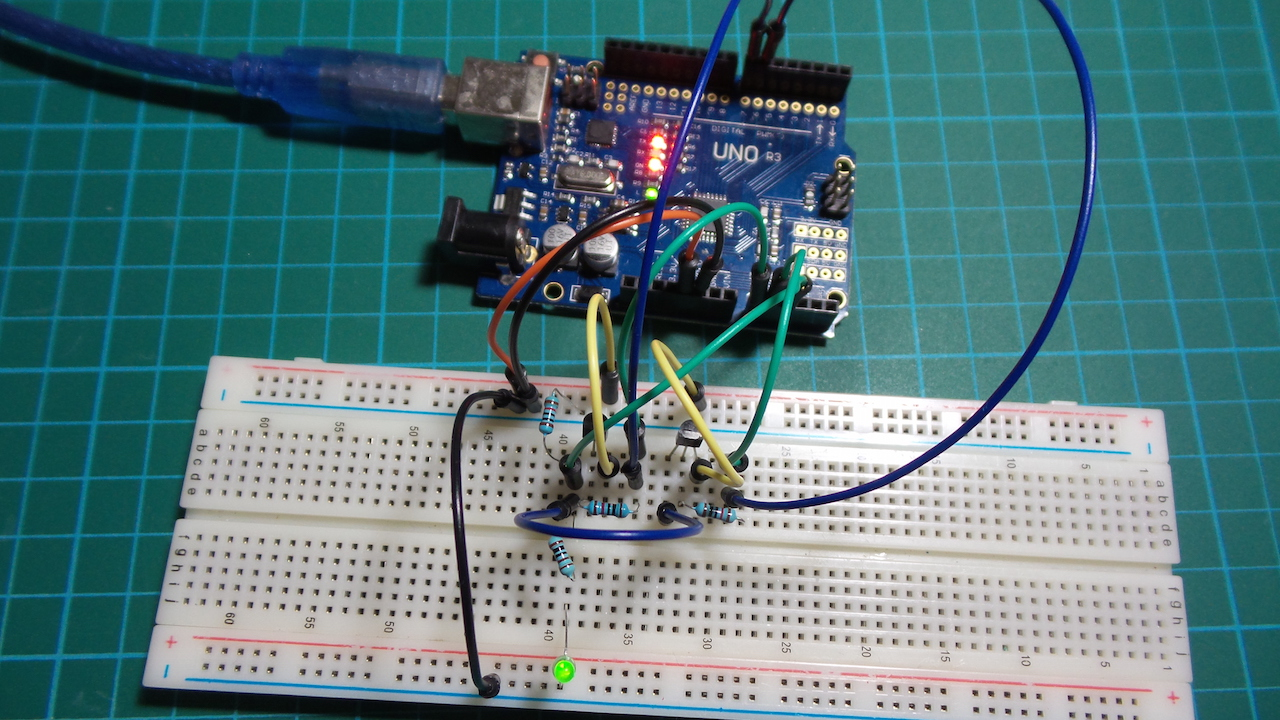# #112 ResistorTransistorLogic/OR

Test the basic resistor-transistor logic OR gate.

Resistor–transistor logic (RTL) is a class of digital circuits built using resistors as the input network and bipolar junction transistors (BJTs) as switching devices. RTL is the earliest class of transistorized digital logic circuit and is largely obsolete now.

It is interesting to see how various logic gate may be realised with BJTs and resistors alone. Here is the basic OR gate.

This is a “classic” two-transistor OR gate, often used when describing the fundamentals of digital logic. Given two ideal transistors (NPN) with common collector, we treat them as switches: when either is on, the output should be high:

A B OUT
0 0 0
0 1 1
1 0 1
1 1 1

Here’s a sample trace. The lower two traces are the signal inputs, and the upper trace is the output of the gate.The Arduino is not really a core part of the circuit. It only does the following:

• provides +5V power supply (for convenience)
• automates the toggling of the driving inputs
• measures the input and output voltages for plotting

## Construction

The circuit uses a digital output pins to sequence inputs to the gate. Three analog input pins are used to read the two input signals and the resuting output, with the values is echoed to the Arduino serial port for plotting with PlotNValues (a simple Processing sketch).